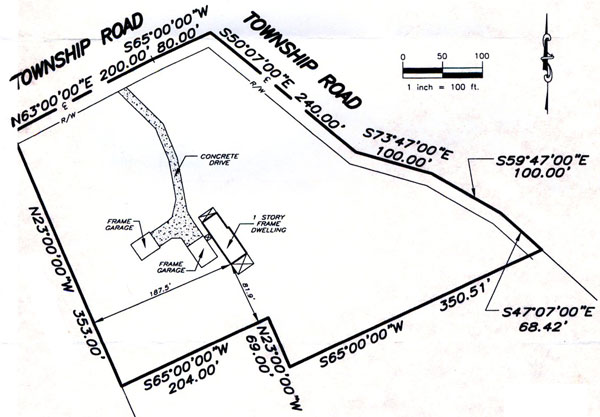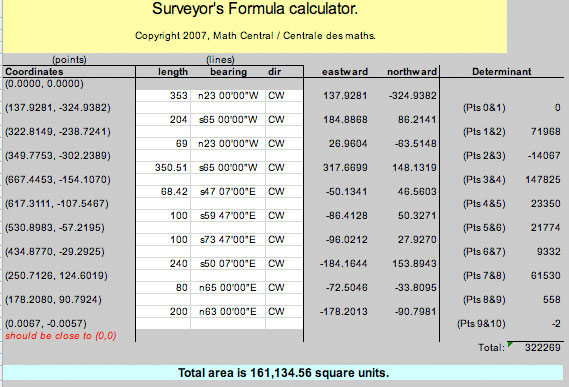SEARCH HOMEMath Central Quandaries & QueriesQuestion from Gregory, a parent: I need to know the area of a six sided piece of property. I sent a map by regular mail. GregHi Greg,

I used the Surveyor's Area formula that Chris Fisher described in an answer to an earlier question and a Microsoft Excel tool the Steve La Rocque constructed to calculate the area. Here is the result from the spread sheet. You will see that I made a change to the survey data. The side of length 80.00 feet should have a bearing of S65o00'00"E.(You can download the spreadsheet in MS excel format if you like).

Your dimensions are in feet so the area is 161,134.56 square feet. There are 43560 square feet in an acre so that's 161,134.56/43560 = 3.7 acres.

HarleyMath Central is supported by the University of Regina and The Pacific Institute for the Mathematical Sciences.